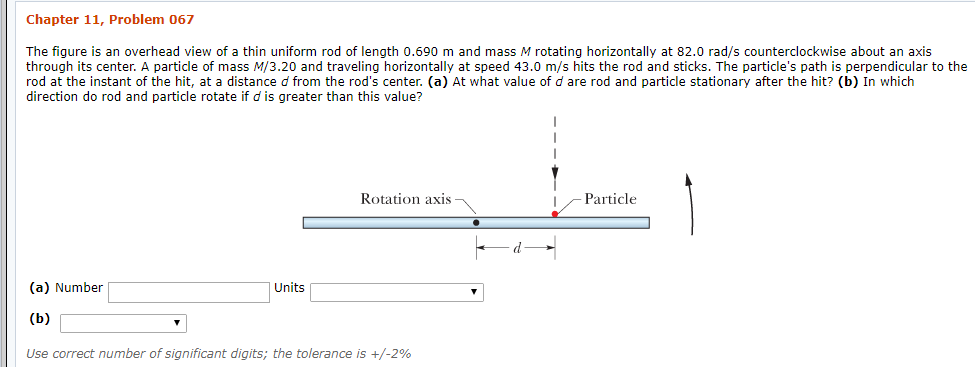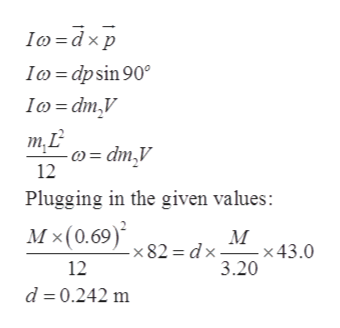# Chapter 11, Problem 067The figure is an overhead view of a thin uniform rod of length 0.690 m and mass M rotating horizontally at 82.0 rad/s counterclockwise about an axisthrough its center. A particle of mass M/3.20 and traveling horizontally at speed 43.0 m/s hits the rod and sticks. The particle's path is perpendicular to therod at the instant of the hit, at a distance d from the rod's center. (a) At what value of d are rod and particle stationary after the hit? (b) In whichdirection do rod and particle rotate if d is greater than this value?Rotation axisParticle(a) NumberUnits(b)Use correct number of significant digits; the tolerance is +/-2%

Questionhelp_outlineImage TranscriptioncloseChapter 11, Problem 067 The figure is an overhead view of a thin uniform rod of length 0.690 m and mass M rotating horizontally at 82.0 rad/s counterclockwise about an axis through its center. A particle of mass M/3.20 and traveling horizontally at speed 43.0 m/s hits the rod and sticks. The particle's path is perpendicular to the rod at the instant of the hit, at a distance d from the rod's center. (a) At what value of d are rod and particle stationary after the hit? (b) In which direction do rod and particle rotate if d is greater than this value? Rotation axis Particle (a) Number Units (b) Use correct number of significant digits; the tolerance is +/-2% fullscreen
check_circleExpert Solution
Step 1

Given values:

Length of the rod, L = 0.69 m

Mass of the rod, m1= M

Angular velocity of the rod, omega = 82 rad/s

Mass of the particle, m2 = M/3.20

Speed of the particle, V = 43.0 m/s

Moment of inertia of the rod about its center, I = ML2/12

Step 2

(a)

The angular momentum theorem:help_outlineImage TranscriptioncloseIo dxp Ico dpsin 90 Io=dm,V т.L -)= dm,V 12 Plugging in the given values: Мx(0.69)* М -x 43 .0 = dx -x 82 12 3.20 d 0.242 m fullscreen
Step 3

(b)

If d is greater the rod wi...

### Want to see the full answer?

See Solution

#### Want to see this answer and more?

Solutions are written by subject experts who are available 24/7. Questions are typically answered within 1 hour*

See Solution
*Response times may vary by subject and question
Tagged in

### Other